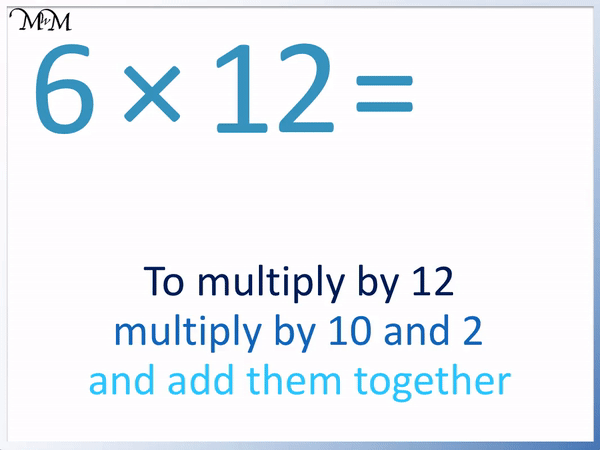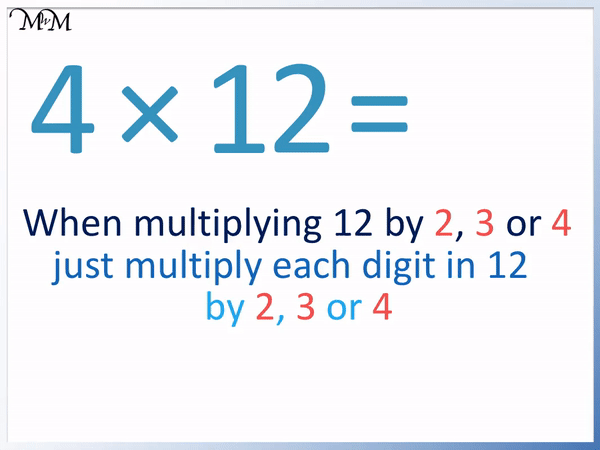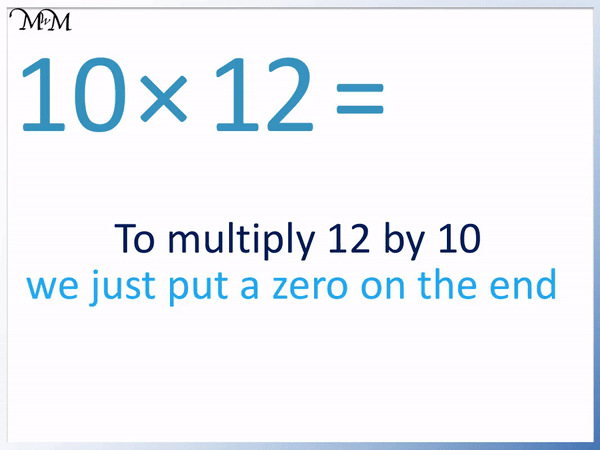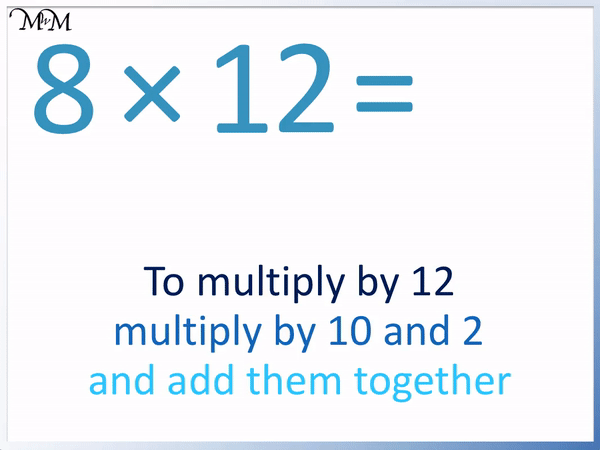# The 12 Times Table

The 12 Times TableThe 12 times table is:

• 1 × 12 = 12
• 2 × 12 = 24.
• 3 × 12 = 36
• 4 × 12 = 48
• 5 × 12 = 60
• 6 × 12 = 72
• 7 × 12 = 84
• 8 × 12 = 96
• 9 × 12 = 108
• 10 × 12 = 120
• 11 × 12 = 132
• 12 × 12 = 144• The 12 times table is made by counting up in twelves.
• To multiply a number by 12, we can multiply the number by 10 and 2 before adding these results.
• Multiplying a whole number by 10 just involves putting a zero on the end.
• Multiplying a number by 2 just involves adding it to itself.
• 6 × 10 = 60 and 6 × 2 = 12.
• 60 + 12 = 72 and so, 6 × 12 = 72.

To multiply a number by 12, multiply it by 10 and 2 then add these results together.• Here is 5 × 12.
• To multiply a number by 12, we multiply by 10 and 2 then add the results together.
• 5 × 10 = 50 and 5 × 2 = 10.
• 50 + 10 = 60 and so, 5 × 12 = 60.#### 12 Times Table Flashcards

Click on the 12 times table flashcards below to memorise the 12 times table.

×
×

=

# The 12 Times Table

## What is the 12 Times Table?

The 12 times table is made by counting up in twelves like so:

• 1 × 12 = 12
• 2 × 12 = 24.
• 3 × 12 = 36
• 4 × 12 = 48
• 5 × 12 = 60
• 6 × 12 = 72
• 7 × 12 = 84
• 8 × 12 = 96
• 9 × 12 = 108
• 10 × 12 = 120
• 11 × 12 = 132
• 12 × 12 = 144

Here is the complete 12 times table chart.## How to Memorise the 12 Times Table

The 12 times table is one of the most difficult times tables to memorise because there are not many patterns and the numbers are relatively large.

The first four numbers in the 12 times table can be memorised since the answers are found by multiplying the digits of 1 and 2 in 12 separately.

For example in 2 × 12, we can simply multiply each digit in 12 by 2.

We multiply the 1 and 2 in 12 by 2.

2 × 1 = 2 and 2 × 2 = 4. Therefore 2 × 12 = 24.Similarly, to find 3 × 12, we multiply the 1 and 2 in 12 by 3.

3 × 1 = 3 and 3 × 2 = 6. Therefore 3 × 12 = 36.Again to find 4 × 12, we multiply the 1 and 2 in 12 by 4.

4 × 1 = 4 and 4 × 2 = 8. Therefore 4 × 12 = 48.The same is true for 1 × 12.

1 × 1 = 1 and 1 × 2 = 2. Therefore 1 × 12 = 12.

However it is easiest to just remember that any number multiplied by 1 does not change. 12 remains as 12 when it is multiplied by 1.This 12 times table trick only works for the first four numbers in the twelve times table.

This is summarised in the table below.One of the easiest numbers in the twelve times table to remember is 10 × 12 = 120.

This is because to multiply a whole number by 10, we simply put a zero on the end.

Putting a zero on the end of 12 gives us 120.For the rest of the 12 times table there are no real easy tricks or patterns. It can be easiest to work out the 12 times table using the following rule:

To multiply by 12, multiply by 10 and 2 and add them together.

This rule is useful because 10 and 2 are relatively easy to multiply by.

We will use this times table rule to work out some numbers in the 12 times table.

For example, here is 5 × 12.

We multiply by 10 and 2 before adding these results together.

5 × 10 = 50 and 5 × 2 = 10.

We add these results to find 5 × 12.

50 + 10 = 60 and so, 5 × 12 = 60.Here is 6 × 12.

We multiply by 10 and 2 ten add the results together.

6 × 10 = 60 and 6 × 2 = 12.

60 + 12 = 72 and so, 6 × 12 = 72.Here is 7 × 12.

7 × 10 = 70 and 7 × 2 = 14.

70 + 14 = 84 and so, 7 × 12 = 84.Here is 8 × 12.

8 ×10 = 80 and 8 × 2 = 16.

80 + 16 = 96 and so, 8 × 12 = 96.In this example we have 9 × 12.

9 × 10 = 90 and 9 × 2 = 18.

90 + 18 = 108 and so, 9 × 12 = 108.It is best to practice these calculations until the answers become familiar and the 12 times table is memorised off by heart.Now try our lesson Prime Numbers to 20 where we learn what prime numbers are.